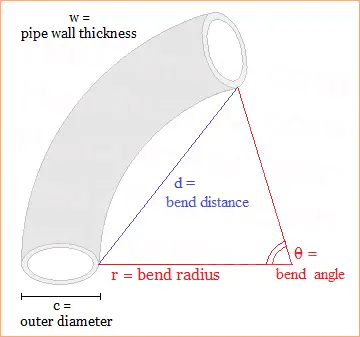# Volume of a Curved Pipe

### Curved Pipe Volume Calculator

Curved Pipe Volume Calculator
Outer Diameter c =
Pipe Thickness w =
Bend Angle θ =
Choose One: Bend Radius r =
Bend Distance d =

Pipe Volume =

Curved pipes in the shape of circular arcs are frequently used in plumbing. To find the capacity of a pipe arc, you can consider the pipe as a section of a torus, and then use the formula for toric volume. When figuring volume of a curved pipe, you must know the outer diameter, thickness, band angle, and either the bend radius OR bend distance. See figure below:Recall that if a torus has a diameter of X and a hole diameter of Y, then the equation for its volume is

Torus Volume = (π2/4)[(X/2)2 - (Y/2)2](X/2 - Y/2)

For a torus sector with an angle measure of θ°, multiply the torus volume by a factor of θ/360. For example, if you have a 90° section of torus, the volume is multiplied by 90/360 or 1/4.

In the case of a curved pipe, we consider the interior of the pipe to be a torus section. If the pipe's width is c, the wall thickness w, and the bend radius r, then the torus diameter X and hole diameter Y are given by the equations

X = 2c - 2w + 2r, or
X/2 = c - w + r
Y = 2r + 2w, or
Y/2 = r + w

Putting all of the pieces together, you get the volume formula for a curved pipe:

Pipe Volume = (π2/4)((c-w+r)2 - (r+w)2)(c-2w)(θ/360)

If you don't know the bend radius, you can calculate it using the bend distance d and the bend angle θ:

r = 0.5d/sin(θ/2)

Example

A curved pipe has a diameter of 4 cm and a wall thickness of 0.3 cm. The bend angle is 72° and the bend distance is 28 cm. Find the volume inside the pipe.

First, we have d = 28 and θ = 72, so the bend radius r = 0.5(28)/sin(72/2) = 23.8182 cm. Next, we use c = 4 and w = 0.3 to find the volume inside the pipe:

Pipe Volume = (π2/4)(27.51822 - 24.11822)(3.4)(72/360)
= (π2/4)(175.5638)(3.4)(0.2)
= 294.5667 cm3